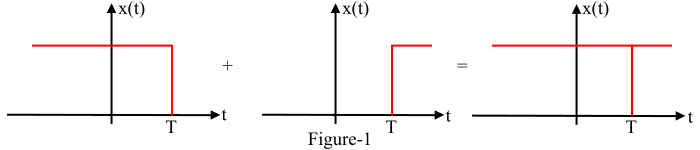# Laplace Transform and Region of Convergence of Two-Sided and Finite Duration Signals

## What is Region of Convergence?

Region of Convergence (ROC) is defined as the set of points in s-plane for which the Laplace transform of a function $\mathrm{\mathit{x\left ( t \right )}}$ converges. In other words, the range of 𝑅𝑒(𝑠) (i.e., 𝜎) for which the function 𝑋(𝑠) converges is called the region of convergence.

## ROC of Two-Sided Signals

A signal $\mathrm{\mathit{x\left ( t \right )}}$ is said to be a two sided signal if it extends from -∞ to +∞. The two sided signal can be represented as the sum of two non-overlapping signals, one of which is right-sided signal and the other is the left-sided signal as shown in Figure- 1.For a two-sided signal, the ROC of the Laplace transform 𝑋(𝑠) is in the form of a strip in the s-plane bounded by two lines 𝜎 = 𝜎r and 𝜎 = 𝜎 i .

## Numerical Example – 1

Find the Laplace transform and ROC of the two-sided signal $\mathrm{\mathit{x\left ( t \right )\mathrm{=}\mathrm{\mathrm{2}}e^{-\mathrm{3}t}u\left ( t \right )\mathrm{+}\mathrm{3}e^{\mathrm{4}t}u\left ( -t \right )}}$

### Solution

The given signal is,

$$\mathrm{\mathit{x\left ( t \right )\mathrm{=}\mathrm{\mathrm{2}}e^{-\mathrm{3}t}u\left ( t \right )\mathrm{+}\mathrm{3}e^{\mathrm{4}t}u\left ( -t \right )}}$$

As the given signal is a two sided signal. The Laplace transform of signal 𝑥(𝑡) is given by,

$$\mathrm{\mathit{L\left [ x\left ( t \right ) \right ]\mathrm{=}\mathrm{2}L\left [ e^{-\mathrm{3}t}u\left ( t \right )\right ]\mathrm{+}\mathrm{3}L\left [ e^{\mathrm{4}t}u\left ( -t \right ) \right ] }}$$

$$\mathrm{\mathit{\Rightarrow X\left ( s \right )\mathrm{=}\frac{\mathrm{2}}{s\mathrm{\mathrm{+}}\mathrm{3}}-\frac{\mathrm{3}}{s-\mathrm{4}};\;\mathrm{ROC} \to Re\left ( s \right )> -\mathrm{3}\: \mathrm{and}\: Re\left ( s \right )< \mathrm{4}}}$$

Therefore, the ROC of the Laplace transform of the signal $\mathrm{\mathit{x\left ( t \right )}}$ is,

$$\mathrm{\mathit{Re\left ( s \right )> -\mathrm{3}\cap Re\left ( s \right )< \mathrm{4}\mathrm{=}-\mathrm{3}< Re\left ( s \right )< \mathrm{4}}}$$The ROC of the Laplace transform of the given signal 𝑥(𝑡) is shown in Figure- 2. It can be seen that the ROC of the given two sided signal is a strip in the s-plane and lies to the right of the pole at 𝑠 = −3 and to the left of the pole at 𝑠 = +4.

## ROC of Finite Duration Signals

A signal $\mathrm{\mathit{x\left ( t \right )}}$ is said to be a finite duration signal if $\mathrm{\mathit{x\left ( t \right )}}$ = 0 for $\mathrm{\mathit{t< T_{\mathrm{1}}}}$ and $\mathrm{\mathit{t> T_{\mathrm{2}}}}$ where $\mathrm{\mathit{T_{\mathrm{1}}}}$ and $\mathrm{\mathit{T_{\mathrm{2}}}}$ are some finite time instants as shown in Figure-3.For a finite duration signal which is absolutely integrable, the ROC of its Laplace transform extends over the entire s-plane.

## Numerical Example – 2

Find the Laplace transform and ROC of the finite duration signal $\mathrm{\mathit{x\left ( t \right )}}$ shown in Figure-4.### Solution

The equation describing the given finite duration signal is given by,

$$\mathrm{\mathit{x\left ( t \right )\mathrm{=}\left [ u\left ( t-T_{\mathrm{1}} \right )-u\left ( t-T_{\mathrm{2}} \right ) \right ]}}$$

Both the terms of this signal are causal. The Laplace transform of this is signal is obtained as −

$$\mathrm{\mathit{L\left [ x\left ( t \right ) \right ]\mathrm{=}L\left [ u\left ( t-T_{\mathrm{1}} \right )\right ]-L\left [ u\left ( t-T_{\mathrm{2}} \right ) \right ] }}$$

$$\mathrm{\mathit{\Rightarrow X\left ( s \right )\mathrm{=}\left ( \frac{e^{-T_{\mathrm{1}}s}}{s}-\frac{e^{-T_{\mathrm{2}}s}}{s} \right )}}$$

$$\mathrm{\mathit{\therefore X\left ( s \right )\mathrm{=}\frac{\mathrm{1}}{s}\left ( {e^{-T_{\mathrm{1}}s}}-{e^{-T_{\mathrm{2}}s}} \right );}\; \; ROC\rightarrow All\; \mathit{s}}$$